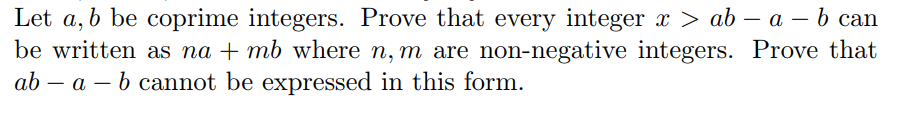Let a, b be coprime integers. Prove that every integer x > ab - a -b canbe written as na 1 mb wher℃ n,m are non negative ini clicrs. Prov℃ thaiab - a b cannot be expressed in this form.

Question

Abstract Algebra. Please explain everything in detail.help_outlineImage TranscriptioncloseLet a, b be coprime integers. Prove that every integer x > ab - a -b can be written as na 1 mb wher℃ n,m are non negative ini clicrs. Prov℃ thai ab - a b cannot be expressed in this form. fullscreen
Step 1

To prove (or disprove)  the possibilty of required representations under the given conditions

Step 2

We work under the given condtions, a, b positive coprime integers, gcd (a,b)=1. By Euclidean algorithm, we know that ANY integer x can be represented as an+bm . The point is that we require that n and m be non-negative integers. To explain this, consider the example a =10,b=3. The statement says that every integer x > 30-10-3=17 can be represented thus. Note that the integer 13 (less than 17) is also of this form , but 17 cannot be represented thus. We need to prove it in the general case.

Step 3

Describing all integral solutions (no conditions) of the equation an+bm =x. The last line expresses all the solutions (...

Want to see the full answer?

See Solution

Want to see this answer and more?

Our solutions are written by experts, many with advanced degrees, and available 24/7

See Solution
Tagged in

Math